• tableau画概率密度、累计概率密度图
千次阅读
2021-02-21 13:48:59

我们要看概率密度或者累计概率密度，参考：

Tableau 快速表计算 显示百分比 / 累计走势

Tableau累计求和指标时间趋势分析

操作步骤如下：

一：将某个数值字段放入维度，因为我们要看的是累计百分比，所以数值字段的具体值就变成了维度

二：创建计算字段，

 累计百分比 百分比 RUNNING_SUM(COUNTD([团长id]))/WINDOW_SUM(COUNTD([团长id])) COUNTD([团长id])/WINDOW_SUM(COUNTD([团长id])) RUNNING_SUM(sum([记录数]))/WINDOW_SUM(sum([记录数])) sum([记录数])/WINDOW_SUM(sum([记录数]))

三：按下图设置字段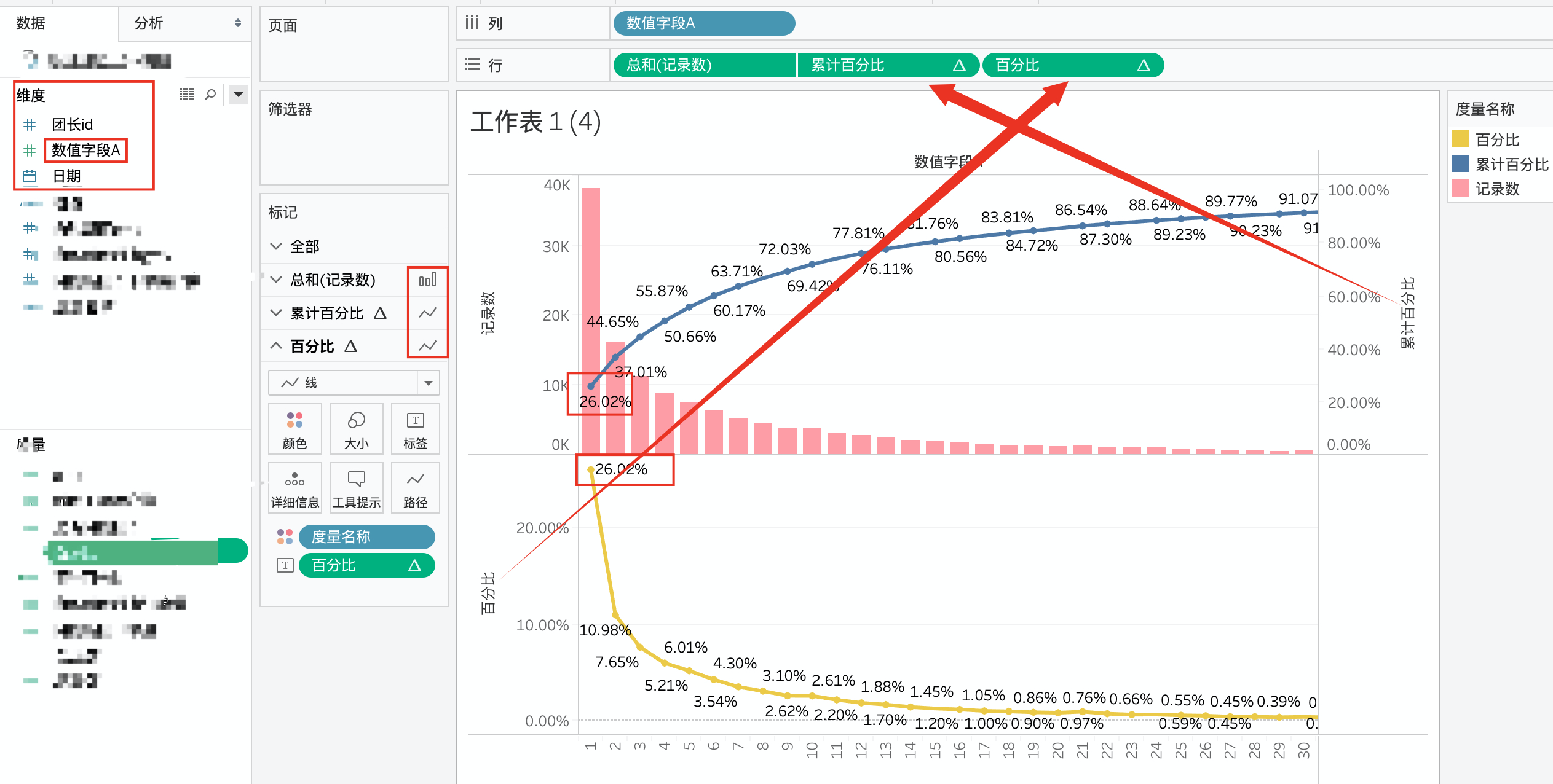更多相关内容
• 目录   1、scipy库中各分布对应的方法   2、stats中各分布的常用方法及其功能   3、正态分布的概率密度函数及其图象 ...    3）python绘制t分布正态分布的概率密度函数对比   6、F分布的概率密度函数
• 对于pandas的dataframe，...对于pandas的dataframe，绘制概率密度图方法如下： //pdf是pandas的dataframe, delta_time是其中一列 pdf.delta_time.dropna().plot(kind='kde', xlim=(-50,300)) 以上这篇python绘制直方
• 用matlab画图像概率密度梯度，基于直方的方法，给大家做个参考吧，^-^

## 1、 导入库

import numpy as np
import pandas as pd
import matplotlib as mpl
import matplotlib.pyplot as plt
import seaborn as sns


## 2、 概率密度图–kdeplot的应用

第一个参数：要绘制的图像数据

# 获得数据
# 获得类别种类
label = np.unique(dataSet.iloc[:,-1])

# 绘制图像
for i in range(len(label)):
data = dataSet.loc[dataSet.iloc[:,-1]==label[i],'age']
data.reset_index(drop=True, inplace=True)
sns.kdeplot(data
#                ,alpha=0.5
,color=plt.cm.tab10(i)
,linewidth=1
,linestyle='-'
,label=label[i]
)
plt.xlabel('x轴')
plt.ylabel('y轴')
plt.title('年龄-客户概率密度');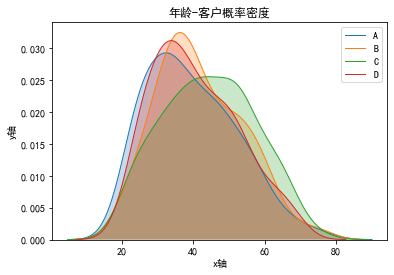## 3、 直方密度图–distplot的应用

参数bins表示对传入的数据分成几组

rdata = np.random.randn(100)
sns.distplot(rdata
,bins=10  #分多少箱，默认8个
,hist=True  #显不显示柱状图
,kde=True  #显不显示密度图
,hist_kws={'histtype':'bar','alpha':0.4}  #柱状图属性,histtype取四种：bar:简单条形图，barstacked:堆积条形图,step:未填充的线图，stepfilled默认被填充的线图
,kde_kws={'color':'g','linestyle':'--','linewidth':1,'alpha':0.7} #密度曲线属性
);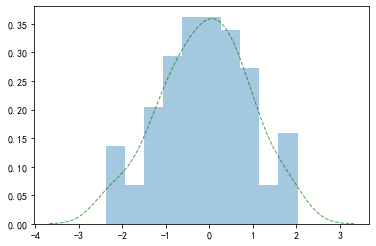## 4、 多条数据

rdata = np.random.randn(100,3)
plt.figure(figsize=(8,4))
for i in range(3):
sns.distplot(rdata[:,i]
,bins=10  #分多少箱，默认8个
,hist=True  #显不显示柱状图
,kde=True  #显不显示密度图
,hist_kws={'color':plt.cm.tab10(i/3),'histtype':'bar','alpha':0.4}  #柱状图属性,histtype取四种：bar:简单条形图，barstacked:堆积条形图,step:未填充的线图，stepfilled默认被填充的线图
,kde_kws={'color':plt.cm.tab10(i/3),'linestyle':'--','linewidth':1,'alpha':0.9} #密度曲线属性
,label=i
)
plt.legend();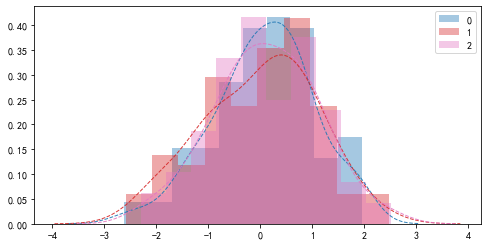展开全文python
• 直方图展示的分布曲线并不平滑，即在一个分组中的样本具有相等的概率密度。 针对直方图的问题，这里提到了核密度图。核密度估计（kernel density estimation）是在概率论中用来估计未知的密度函数，属于非参数检验...
• 1. 直方图和概率密度图叠加 #python 画概率密度图 #-*- coding: utf-8 -*- import matplotlib.pyplot as plt import numpy as np import seaborn as sns # 1）准备数据 lengths = [] with open("D:/length_analysis...

害，折腾了我好久

## 1. 直方图和概率密度图叠加

#python 画概率密度图
#-*- coding: utf-8 -*-
import matplotlib.pyplot as plt
import numpy as np
import seaborn as sns

# 1）准备数据
lengths = []
with open("D:/length_analysis.tsv","r") as f:
for l in f:
if int(l.split('\t'))>80:
continue
lengths.append(int(l.split('\t'))//2)

# 2）设置内置背景style
plt.style.use('seaborn')
# 添加网格显示
plt.grid(linestyle="-", alpha=0.5,linewidth=1.5)

# 3）画图
bins=[0,5,10,15,20,25,30,35,40]
sns.distplot(lengths, bins,hist=True,kde=True,color='royalblue')

# 4）调整
# 修改x轴刻度显示
plt.xticks(range(0, 45)[::5] ,fontsize=10)
# 修改刻度值大小
plt.tick_params(labelsize=13)
# # 添加x, y轴描述信息
# # plt.xlabel("")
# # plt.ylabel("")

plt.show()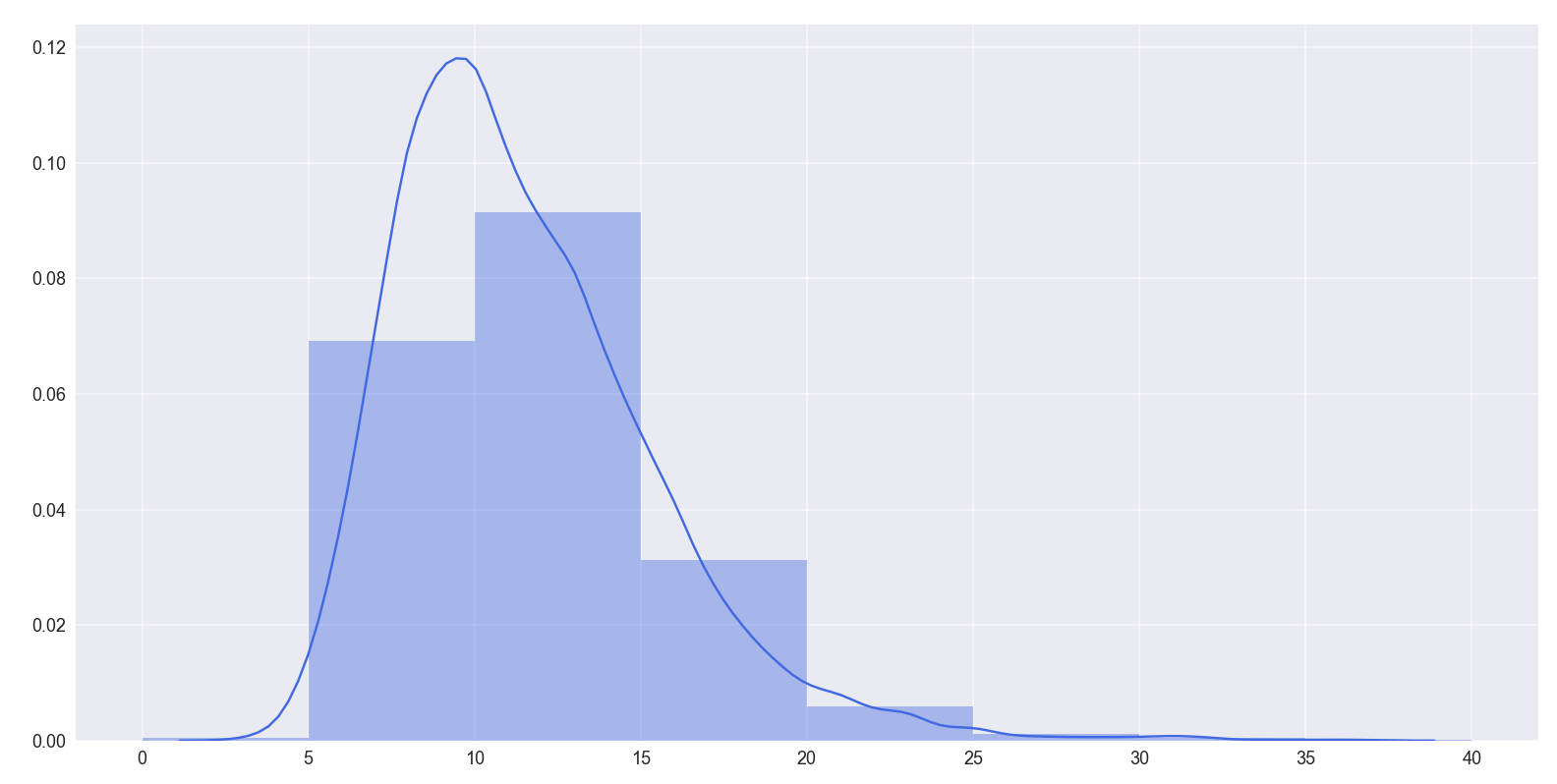## 2. 折线图

import matplotlib.pyplot as plt

# 2）设置内置背景style
plt.style.use('seaborn')
# 添加网格显示
plt.grid(linestyle="-", alpha=0.5,linewidth=1.5)

#折线图
x = [u'<=5',u'5<l<=10',u'10<l<=15',u'15<l<=20',u'20<l<=25',u'25<l<=30',u'30<l<=35',u'35<l']#点的横坐标
k1 = [0.909090909, 0.853952395, 0.84803377, 0.834743006, 0.789830508, 0.773584906, 0.625, 0.285714286]#线1的纵坐标
k2 = [0.909090909, 0.888627681, 0.872250611, 0.860767729, 0.850847458, 0.849056604, 0.583333333, 0.714285714]#线2的纵坐标
k3 = [0.909090909, 0.889509257, 0.884692291, 0.869225764, 0.861016949, 0.849056604, 0.708333333, 0.571428571]
for i in range(len(k1)):
k1[i]*=100
k2[i]*=100
k3[i]*=100

plt.plot(x,k3,'s-',color = 'red',label="Bert + MS-SAN")#o-:圆形
plt.plot(x,k2,'o-',color = 'royalblue',label="MS-SAN")#o-:圆形
plt.plot(x,k1,'*-',color = 'limegreen',label="Bert-base")#s-:方形

plt.tick_params(labelsize=12)
plt.xlabel("Avg_length(l)")#横坐标名字
plt.ylabel("ACC(%)")#纵坐标名字
plt.legend(loc = "best")#图例
plt.show()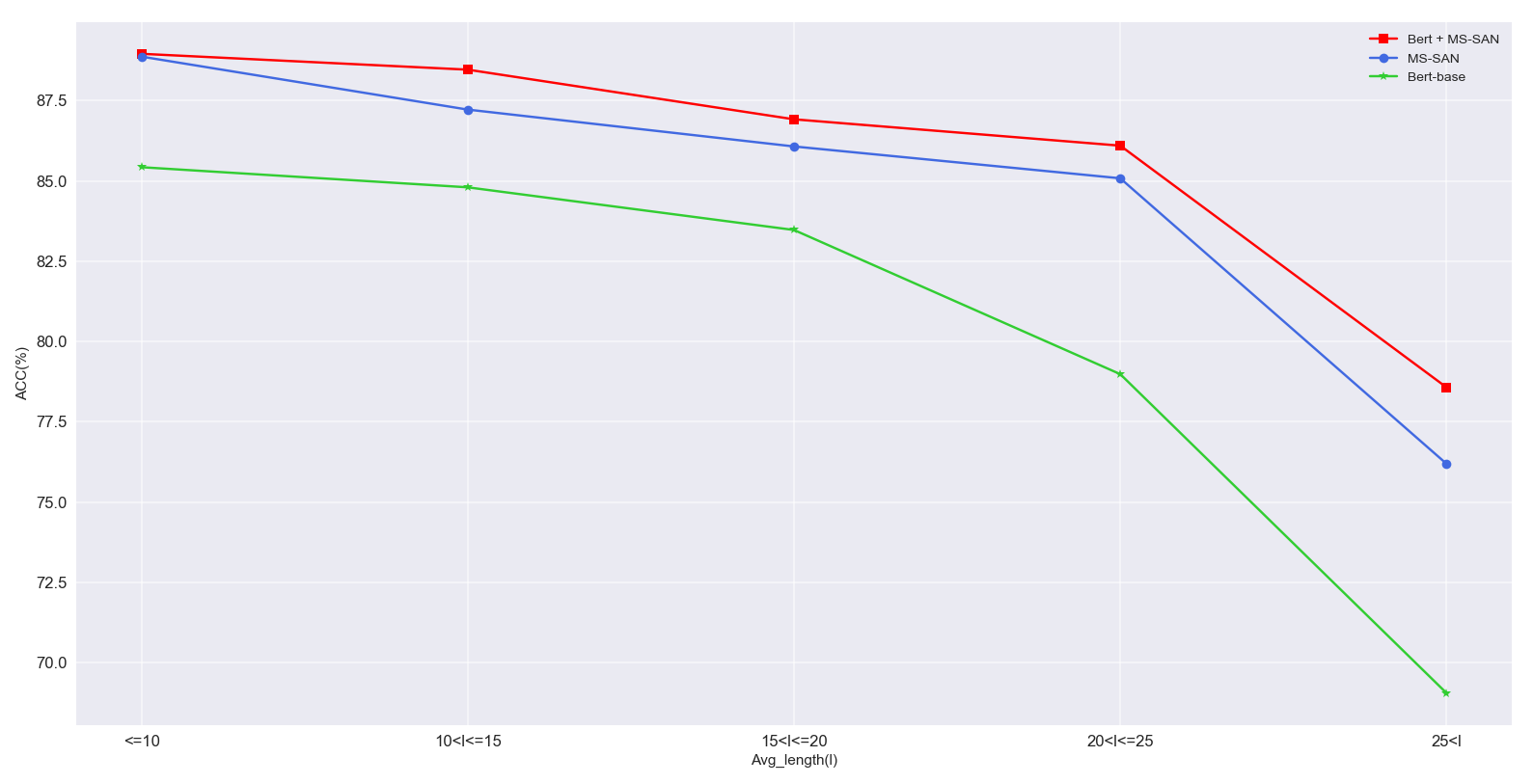补充：

1. mark
'.'       point marker
','       pixel marker
'o'       circle marker
'v'       triangle_down marker
'^'       triangle_up marker
'<'       triangle_left marker
'>'       triangle_right marker
'1'       tri_down marker
'2'       tri_up marker
'3'       tri_left marker
'4'       tri_right marker
's'       square marker
'p'       pentagon marker
'*'       star marker
'h'       hexagon1 marker
'H'       hexagon2 marker
'+'       plus marker
'x'       x marker
'D'       diamond marker
'd'       thin_diamond marker
'|'       vline marker
'_'       hline marker


2, color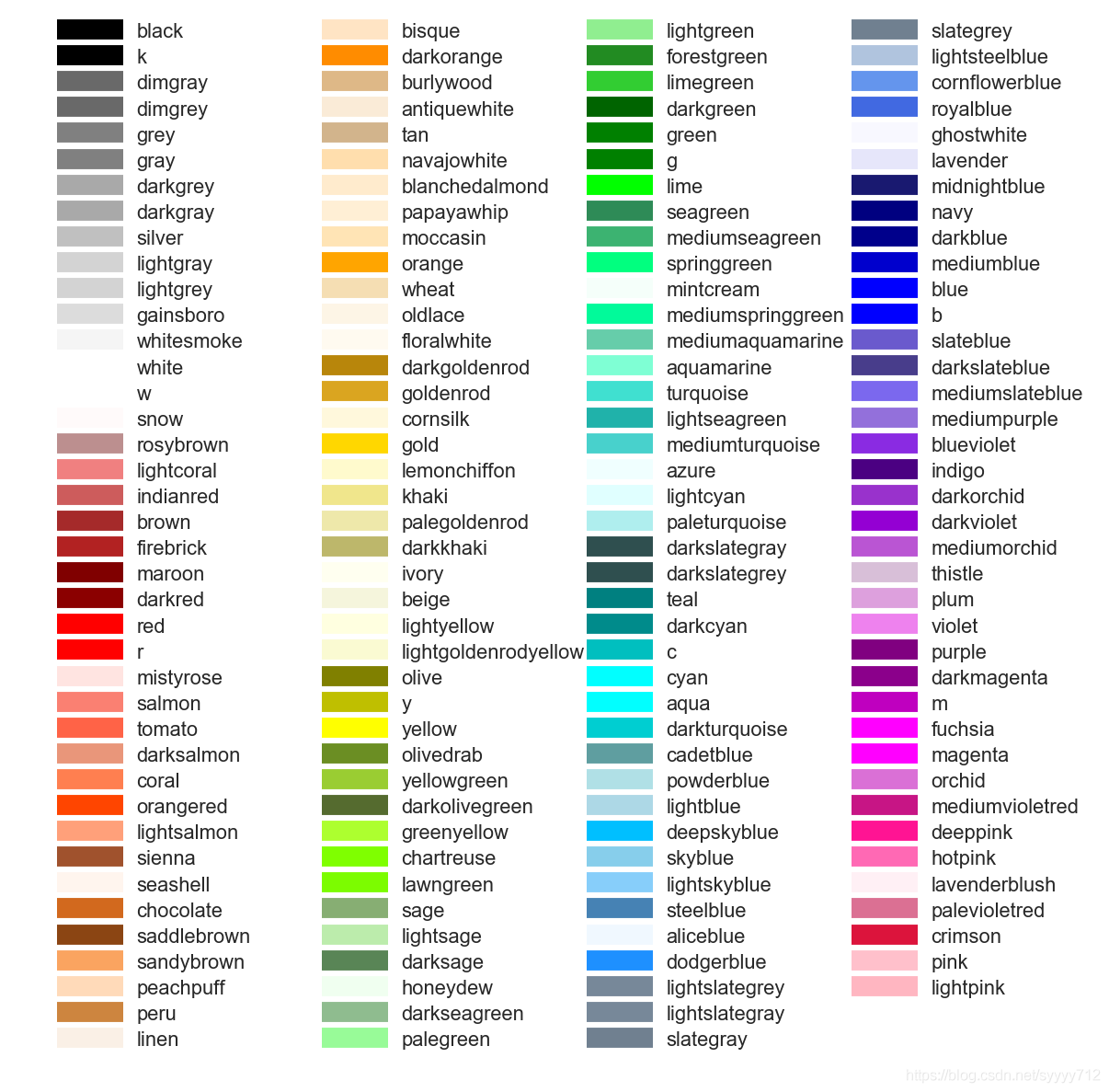展开全文python
• matlab开发-直方拟合概率密度计数频率。输出数据的已清理版本的HistFit
• 由于分析数据的需要，研究了一下正态分布图，经过在网上的总结，自己通过Excel绘制出了正态分布图，实际也可以叫正态分布概率密度图，正态分布图里面包含着积分学和概率学知识，大学学的东西到现在已经还给老师了，...
• 该函数制作归一化直方，即概率密度函数的估计。 直方的面积等于 1，因为该面积低于理论 PDF 函数。 您可以使用此脚本的输出将经验数据与特定分布的理论 PDF 进行比较。 输入： 数据 - 经验数据bins - 直方 ...matlab
• 1 bins=4的概率密度直方 2 bins=40的概率密度直方 3 bins=4的累积分布直方 4 bins=40的累积分布直方 5 bins等于样本总数时的概率密度直方 6 bins等于样本总数时的累积分布直方 下面是绘图...
• 在概率密度直方图中，取到任一bin中的一个样本的平均概率，等于该箱的高度(纵坐标y值) × 宽度(横坐标间距δx) ÷ 该箱中样本个数(n_samples)，而不是等于纵坐标y值；即此时面积表示概率之和，而不是纵坐标y值表示单一样本概率；如图1、图2所示。
• 在累积分布直方图中，取到任一bin中的一个样本的平均概率，等于（该箱的高度(纵坐标y值) －左侧箱的高度）/ 该箱中样本个数；即此时纵坐标y值表示概率，但为累计概率；如图3、图4所示。
• 当箱的个数等于样本总数，即每个箱中只有一个样本时，取到任意一个样本的概率，在概率密度直方图中，等于该箱的高度 × 宽度；在累积分布直方图中，等于该箱的高度－左侧箱的高度。此时累积分布直方图趋近于累计分布函数(CDF)，但概率密度直方图中各箱顶点的连线通常并不趋近于概率密度函数(PDF)，因为在各个横坐标处，也就是样本的取值处，通常会存在离群的样本取值概率，也就是离群的纵坐标值；只有对该直方图取一定程度的平滑曲线，才趋近于概率密度函数；如图5、图6所示。

图1 bins=4的概率密度直方图图2 bins=40的概率密度直方图图3 bins=4的累积分布直方图图4 bins=40的累积分布直方图图5 bins等于样本总数时的概率密度直方图图6 bins等于样本总数时的累积分布直方图• 下面是绘图的代码：
import numpy as np
import matplotlib.pyplot as plt
from sklearn.datasets import make_regression

X, y_origin = make_regression(n_samples=10000, noise=100, random_state=0)  # create data
y_scale = (y_origin + abs(y_origin.min())) / 200  # shift and shrink
y_exp = np.expm1(y_scale)  # exp(x) - 1
y_log = np.log1p(y_exp)  # log(1 + x)
print(sum(y_scale - y_log < 1e-10) == len(y_origin))  # y_log is equal to y_scale

n_samples = 2500  # each bin has n_samples, so there are len(y_origin) / n_samples bins.

f, (ax0, ax1) = plt.subplots(1, 2)
ax0.hist(y_origin, bins=int(len(y_origin)/n_samples), density=True, cumulative=True)
ax0.set_title('y_origin')
ax1.hist(y_scale, bins=int(len(y_scale)/n_samples), density=True, cumulative=True)
ax1.set_title('y_scale')
f.suptitle("Synthetic data", y=0.06, x=0.53)
f.tight_layout(rect=[0.05, 0.05, 0.95, 0.95])
plt.show()

f, (ax0, ax1) = plt.subplots(1, 2)
ax0.hist(y_exp, bins=int(len(y_exp)/n_samples), density=True, cumulative=True)
# ax0.set_xlim([-100, 1700])
ax0.set_title('y_exp')
ax1.hist(y_log, bins=int(len(y_log)/n_samples), density=True, cumulative=True)
ax1.set_title('y_log')
f.suptitle("Synthetic data", y=0.06, x=0.53)
f.tight_layout(rect=[0.05, 0.05, 0.95, 0.95])
plt.show()

展开全文累积分布
• 二维高斯分布概率密度函数数据集实战优化坐标轴与图像优化图像再次优化 概率密度函数 大家肯定都有听说过正态分布，其实正态分布只是概率密度分布的一种，正态分布的概率密度函数均值为μ ，标准差σ是高斯函数的一...
• #matplotlib inline #IPython notebook中的魔法方法，这样每次运行后可以直接得到图像，不再需要使用plt.show() import numpy as np #导入numpy包，用于生成数组 import seaborn as sns #习惯上简写成sns ...python 开发语言 后端
• 我正在研究一个由“脉冲长度”值对应于每个脉冲长度的5或6个“电压值”组成的数据集。第一个值是脉冲长度，后面是电压。找到下表。在15-56伏-47伏-53伏-50伏-50伏16-49伏-46伏-52伏-47伏-50伏47伏-50伏-50伏18-50...
• matlab作概率密度函数很简单，但是本人学的python，因此想用python作图，从txt文本中读取数据，然后用python作概率密度函数图像，
• for i in sorted(list(set(df['yearmonth']))): df_sort = df[df['yearmonth'] == i].groupby('cust_id')['y','pred'].sum().sort_values('y',ascending = False) df_sort['yearmonth'] = i ...python
• ## 绘制概率密度

千次阅读 2021-02-04 07:12:24
最常见的方法是使用fill_between对置信区间之间的区域进行着色。例如：import numpy as npnp.random.seed(1977)import matplotlib.pyplot as plt# Generate data...x_obs = np.linspace(-2, 2, 20)true_model = [0.2...
• 主要介绍了python 计算概率密度、累计分布、逆函数的例子,具有很好的参考价值，希望对大家有所帮助。一起跟随小编过来看看吧
• 针对高斯混合概率假设密度SLAM(GMPHD-SLAM) 算法存在的估计精度低计算代价高的问题, 提出一种无迹高斯混合概率假设密度SLAM算法(unscented-GMPHD-SLAM). 其主要特点在于: 将无迹卡尔曼滤波器应用于机器人位姿粒子...
• % To generate N realizations of a zero-mean unit-variable % Gauss N=100; X1=randn(N,1); [countsX_g,centersX_g]=hist(X1,20); figure(1) subplot(3,1,1) bar(countsX_g);...X2=2*sqrt(3)*(rand(N,1)-0.5);...matlab
• ## 统计学中常见的概率密度图像

万次阅读 多人点赞 2018-09-01 11:43:57
统计学中常见的概率密度图像 今天复习概率论与数理统计看到常见的几种分布突然有了兴趣，所以尝试着写一写关于matlab画出常见几种概率密度图像（注：有些是从网上搜集整理得到的） 二项分： 二项分布,又称n重伯...
• matlab代码如下：number=10000 data=randn(number,1); [y,x]=hist(data,100); %分为100个区间统计，(你可以改你需要的区间数) ... %计算概率密度 ，频数除以数据种数，除以组距 y=y/number figure(1) bar(x,y,1); ...
• 首先基于仿射-尺度不变特征转换（ASIFT）算法，利用亮度概率密度梯度进行兴趣点提取；然后将提取后的兴趣点映射回原图像，构建颜色直方作为图像的特征；最后通过计算不同图像特征间的相似度进行图像检索。仿真实验...
• kde（核密度估计）是对未知分布的估计，所以可能有超出去值范围的估计（比如下我定义的有效范围是0-180，但kde在<0>180时仍然有曲线）。只要看自己想要的范围就好了，它在上面的拟合是可以的。 当然，也...python
• 分别画出了模数转换后信号的前128点、这128个点的直方图和所有采样点得到的直方。很明显直方中表示了每个采样值出现的次数，当采样数越多时曲线越平滑。 根据直方的定义，直方中所有值之等于信
• 利用MATLAB进行随机信号高斯信号的生成及概率密度函数PDF的分析
•数学
• 这些参数可以通过观测样本借助最大似然理论以及贝叶斯估计来确定，被称为概率密度建模的参数化方法。局限性：可能刚开始对样本数据集的概率分布的判断错误，导致其概率模型的表现很差，比如某数据过程是多峰的，高斯...
• 图像颜色空间转换及matlab实现、图像噪声概率密度分布与MATLAB实现、理想滤波器设计与MATLAB实现、巴特沃斯滤波器设计与MATLAB实现、高斯滤波器设计与MATLAB实现...